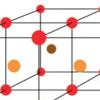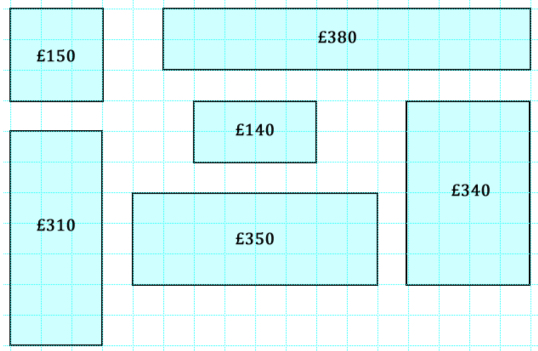#### You may also likeThink of a number and follow the machine's instructions... I know what your number is! Can you explain how I know?### Diagonal Sums

In this 100 square, look at the green square which contains the numbers 2, 3, 12 and 13. What is the sum of the numbers that are diagonally opposite each other? What do you notice?### Finding 3D Stacks

Can you find a way of counting the spheres in these arrangements?

# Price Match

## Price Match

You might like to try Through the Window before this challenge.

”‹In a particular shop, the price of windows is calculated according to the area of glass used and the length of frame needed.

In the picture below, glass costs  £10 for an area of one small square, plus £5 for every square edge of frame.If we call the area of the glass A and the perimeter of the window P, how would you express the cost of the window in terms of A and P?

Use your formula to find pairs of rectangular windows that are different sizes but cost the same.

### Why do this activity?

This activity would make a good introduction to creating and using formula.  It can be approached in a variety of ways, depending on the experience of the learners, and does not necessarily require an ability to manipulate expressions algebraically. It lends itself to a combination of trial and improvement, and working systematically.

### Possible approach

It would be worth projecting the image for the group to see and sharing the information about how the cost of windows is calculated.  Give learners some time (in pairs) to check that they understand the pricing structure, perhaps by testing that a few of the windows are priced correctly.

Invite some pairs to share how they went about this checking process and then introduce the challenge of finding a formula to express the total cost.  You may find that some pairs have already generalised in this way so it could be a case of building on some contributions and working on this part of the problem as a whole group.  (It is not necessary that every pair has expressed the formula in exactly the same way, but you may want to have a conversation about how we know they are equivalent.)

The main part of the task might be best tackled in small groups.  You could have a mini plenary after a short time to share ways of working and you may find that some groups adopt different approaches as a result.

The problem does not specifically ask learners to find all the pairs of windows which have the same cost but a plenary could focus on how you could guarantee that you do not miss any solutions.